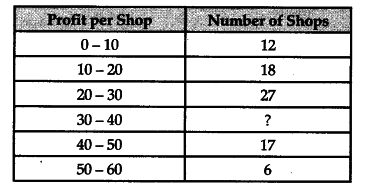# Calculate the number of shops corresponding to class interval 30 - 40 of the following distribution

Calculate the number of shops corresponding to class interval 30 - 40 of the following distribution.The mean profit per shop is 28.

As per the above question, number of shops corresponding to class interval 30 - 40 is missing and mean profit per shop is 28. To calculate missing frequency, we will use the step deviation method of Arithmetic Mean.Let/be the missing frequency.